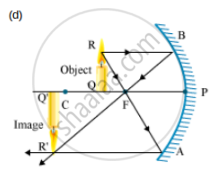# A Student Wants to Project the Image of a Candle Flame on a Screen 60 Cm in Front of a Mirror by Keeping the Flame at a Distance of 15 Cm from Its Pole. - Science

A student wants to project the image of a candle flame on a screen 60 cm in front of a mirror by keeping the flame at a distance of 15 cm from its pole.

(a) Write the type of mirror he should use.

(b) Find the linear magnification of the image produced.

(c) What is the distance between the object and its image?

(d) Draw a ray diagram to show the image formation in this case.

#### Solution

(a) He should use a concave mirror, as it forms a real image on the same side of the mirror.

(b) Object distance, u = -15 cm

Image distance, v = - 60 cm

"Magnification, "m=-v/u=-((-60))/((-15))=-4" cm"

The minus sign in magnification shows that the image formed is real and inverted.

(c) The image is formed at a distance of 45 cm from the object.In this case, the image is formed beyond the centre of curvature. This image is real, inverted and enlarged.

Concept: Magnification Due to Spherical Lenses
Is there an error in this question or solution?
2013-2014 (March) All India Set 1

Share